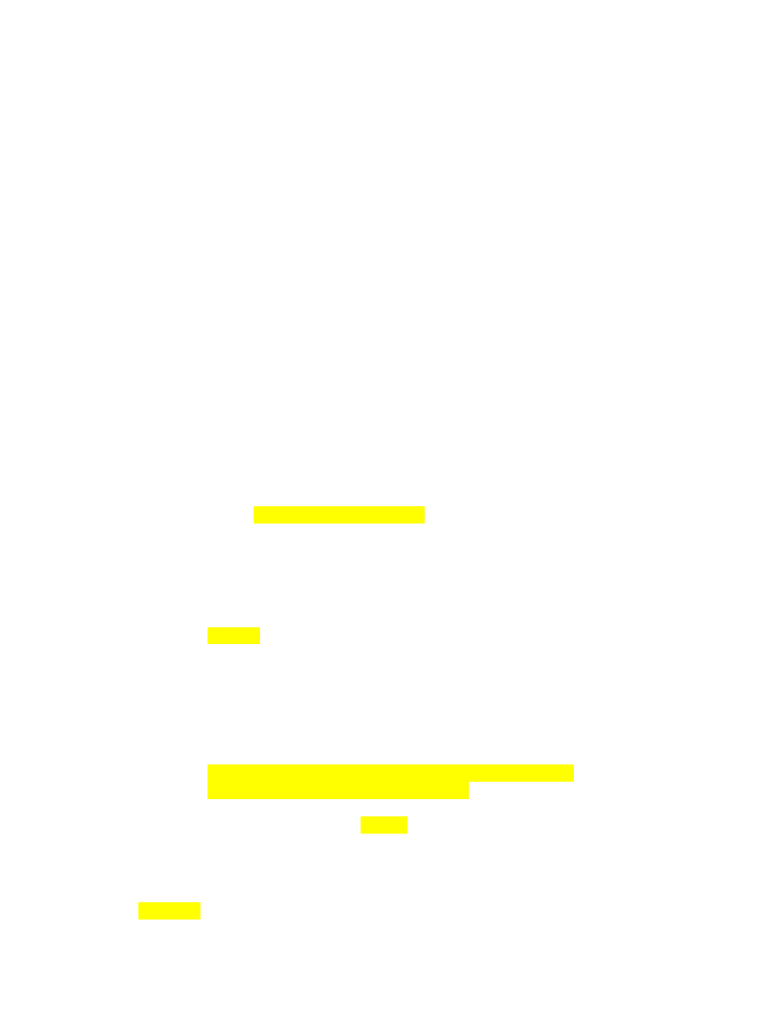# BU121 Lecture Notes - Monocle, Fixed Cost, Top Hat

38 views3 pages
School
Department
Course
ProfessorRecap of Key Concepts So far
Cash is the currency of business
-businesses that show a profit can still go bankrupt
Where profit does not = cash
Creating a statement of cash flows
o Adjust profit to show impact on cash (don’t memorize but instead try to understand it
because there is too much to memorize)
Cash survival breakeven is when EBDAT = 0
Calculate EBDAT
(tells you a push before you breakeven)
Calculating breakeven
Driving break even
Creating a cash budget
o Developing worksheet to manage timing of cash inflows and out flows
o Format (you need to memorize the formula for the break even point)
o Process (do they need to borrow or pay back money by the end of the month)
Top hat monocle question
1. Cash and profit are not the same thing because
-depreciation is non cash item
-sales revenue on the income statement is not necessarily received
2. Which of the following would have a positive impact on cash
Receivable
Payable when it increases
Gross fixed impact
3. which of the following has a negative impact on cash
a. decrease in receivables
b. increase in payable
c. increase in common stock
d. increase in long term liability
e. none
4. which of the following best describe what drives the level of sale a firm needs to break even
cash back
a. level of fixed cost including depreciation
b. variable cost revenue ratio
c. contribution margin
d. survival revenue
e. ebdat
f. b and c only (because b affects c and c is what you focus on)
revenue variable cost = contribution margin
5. if a company has cash fixed costs of \$100 000 revenue of \$400 000 and cost of goods sold of
\$200 000 what is its cash break even? 200,000 every sale you need to cover variable cost
therefore you divide it into fixed cost
6. if a company has revenue of \$500 000, cost of goods sold \$300 000, fixed costs of 150 000
including depreciation of \$10 000 and interest of \$20, 000 what is its EBDAT
a. 200 000
b. 40 000
Unlock document

This preview shows page 1 of the document.
Unlock all 3 pages and 3 million more documents.

## Document Summary

Businesses that show a profit can still go bankrupt. Creating a statement of cash flows: adjust profit to show impact on cash (don"t memorize but instead try to understand it because there is too much to memorize) Cash survival breakeven is when ebdat = 0. Calculate ebdat (tells you a push before you breakeven) Top hat monocle question: cash and profit are not the same thing because. Sales revenue on the income statement is not necessarily received: which of the following would have a positive impact on cash. All of cash expenses covered therefore 500, 000 300 000 = 200 000 where 200 000 150. 000 = 50 000 10 000 = 40 000: you forecast your sales revenue for january of 000. 20 because 4000 time 6% and divide it by months. To be liquid you need to generate enough cash to pay bills (problem is you use money to invest inorder to make money)

# Get access

\$10 USD/m
Billed \$120 USD annually
Homework Help
Class Notes
Textbook Notes